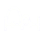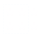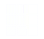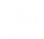# Interactive Problem Bank

•Fraction Circles
•Number Lines
•Fraction Bars
•Sets
•Area Models
•Base Ten Blocks

## Analyzing Proportional Relationships

• Students represent proportional and non-proportional situations using diagrams, tables, coordinate plane, equations, and real-world situations.
• Students visualize relationships between quantities and recognize if they are proportional or non-proportional.
• Students explore a variety of representations to determine if a situation is proportional or not.